JEE  >  JEE Advanced (Subjective Type Questions): Solutions- 2

# JEE Advanced (Subjective Type Questions): Solutions- 2 - Chemistry 35 Year Past year Papers JEE Main & Advanced

7. The vapour pressure of ethanol and methanol are 44.5 mm and 88.7 Hg respectively. An ideal solution is formed at the same temperature by mixing 60 g of ethanol with 40 g of methanol. Calculate the total vapour pressure of the solution and the mole fraction of methanol in the vapour. (1986 - 4 Marks)

Ans : 66.17 mm, 0.65

Solution :
TIPS/Formulae :
Ptotal = pA + pB
Molecular weight of CH3OH = 12 + 3 + 16 + 1 = 32
Molecular weight of C2H5OH= 24 + 5 + 16 + 1 = 46
According to Raoult’s law
Ptotal = p1 + p2
where Ptotal = Total vapour pressure of the solution
p1 = Partial vapour pressure of one component
p2 = Partial vapour pressure of other component
Again, p1 = Vapour pressure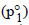× mole fraction
Similarly, p2 = Vapour pressure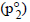x mole fraction

Mole fraction of CH3OH =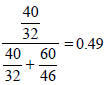Mole fraction of ethanol =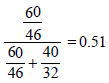NOTE THIS STEP : Thus now let us first calculate the partial vapour pressures, i.e., p1 and p2 of the two component.
Partial vapour pressure of CH3OH(p1)= 88.7 × 0.49 = 43.48 mm
Partial vapour pressure of C2H5OH(p2) = 44.5 × 0.51 = 22.69 mm
∴ Total vapour pressure of the solution = 43.48 + 22.69 mm = 66.17 mm
Mole fraction of CH3OH in vapour =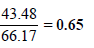8. The vapour pressure of a dilute aqueous solution of glucose (C6H12O6) is 750 mm of mercury at 373 K. Calculate (i) molality and (ii) mole fraction of the solution. (1989 - 3 Marks)

Ans : 0.7503 mol/kg, 0.9868

Solution :
TIPS/Formulae :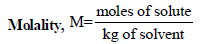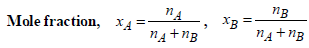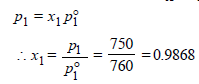x2 (solute) = 1 – 0.9868 = 0.0132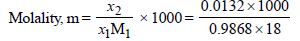= 0.7503 mol kg–1

9. The vapour pressure of pure benzene at a certain temperature is 640 mm Hg. A non-volatile non-electrolyte solid weighing 2.175 g is added to 39.0 g of benzene. The vapour pressure of the solution is 600 mm Hg. What is the molecular weight of the solid substance? (1990 - 3 Marks)

Ans : 65.25

Solution :
TIPS/Formulae :

According to Raoult’s law,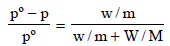Here, pº = 640 mm
p = 600 mm
w= 2.175 g
= 39.0
m = ?
M = 78
Substituting the various values in the above equation for Roult's law :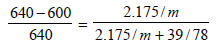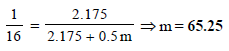10. The degree of dissociation of calcium nitrate in a dilute aqueous solution, containing 7.0 g. of the salt per 100 gm of water at 100ºC is 70%. If the vapour pressure of water at 100ºC is 760 mm, calculate the vapour pressure of the solution. (1991 - 4 Marks)

Ans : 746.3 mm Hg

Solution :
TIPS/Formulae : First find moles of Ca(NO3)2 and water.
Then use the expression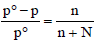to find vapour pressure of solution.
Let initially 1 mole of Ca(NO3)2 is taken
Degree of dissociation of Ca(NO3)2 = 70/100 = 0.7
Ionisation of Ca(NO3)2 can be represented as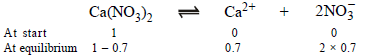∴ Total number of moles in the solution at equilibrium = (1 – 0.7) + 0.7 + 2 × 0.7 = 2.4

No. of moles when the solution contains 1 gm of calcium nitrate instead of 1 mole of the salt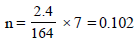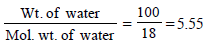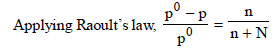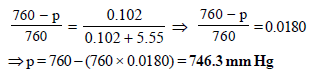11. Addition of 0.643 g of a compound to 50 ml. of benzene (density : 0.879 g/ml.) lowers the freezing point from 5.51ºC to 5.03ºC. If Kf for benzene is 5.12 K kg mol–1, calculate the molecular weight of the compound. (1992 - 2 Marks)

Ans : 156.056

Solution :

TIPS/Formulae :
Given Wt. of benzene (solvent),
W = Volume × density = 50 × 0.879 = 43.95 g
Wt. of compound (solute), w = 0.643 g
Mol. wt. of benzene, M = 78; Mol. wt. of solute, m = ?
Depression in freezing point, DTf = 5.51 – 5.03 = 0.48
Molal freezing constant, Kf = 5.12
Now we know that,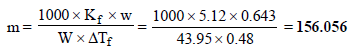12. What weight of the non-volatile solute, urea (NH2 – CO – NH2) needs to be dissolved in 100g of water, in order to decrease the vapour pressure of water by 25%? What will be the molality of the solution? (1993 - 3 Marks)

Ans : 18.52 m

Solution :

TIPS/Formulae :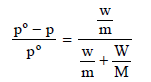Here, w and m are wt. and molecular wt. of solute, W and M are wt. and molecular weight of solvent
p = Pressure of solution; pº = Normal vapour pressure
Let the initial (normal) pressure (pº) = p

∴ Pressure of solution =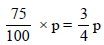m = 60, M = 18, W = 100 gm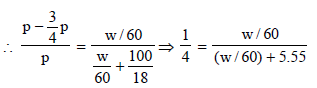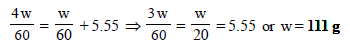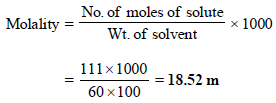13. The molar volume of liquid benzene (density=0.877 g mL–1) increases by a factor of 2750 as it vaporises at 20°C and that of liquid toluene   (density=0.867 g mL–1) increases by a factor of 7720 at 20°C. A solution of benzene and toluene at 20°C has a vapour pressure of 46.0 Torr. Find the mole fraction of benzene in the vapour above the solution. (1996 - 3 Marks)
Ans : 0.73

Solution :

TIPS/Formulae :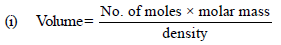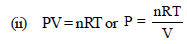Volume of 1 mole of liq. benzene =78/0.877
Volume of 1 mole of toluene = 92/0.867

In vapour phase,
At 20°C, for 1 mole of benzene,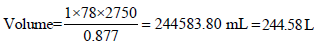Similarly for 1mole of toluene,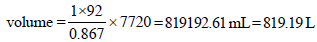As we know that, PV = nRT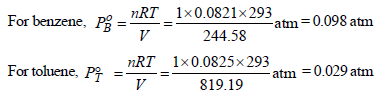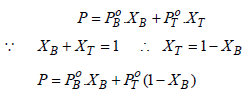Total vapour-pressure = 46 torr = 46/760 = 0.060  atm
Thus, 0.060 = 0.098 XB + 0.029 (1 – XB)
⇒ 0.060 = 0.098 XB + 0.029 – 0.029 XB
⇒ 0.031 = 0.069 XB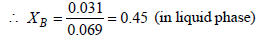XB + XT = 1
XT = 1– 0.45 = 0.55 (in liquid phase)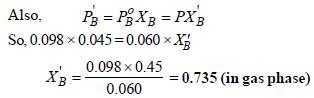The document JEE Advanced (Subjective Type Questions): Solutions- 2 | Chemistry 35 Year Past year Papers JEE Main & Advanced is a part of the JEE Course Chemistry 35 Year Past year Papers JEE Main & Advanced.
All you need of JEE at this link: JEE

## Chemistry 35 Year Past year Papers JEE Main & Advanced

142 docs|66 tests

## Chemistry 35 Year Past year Papers JEE Main & Advanced

142 docs|66 testsExplore Courses for JEE examSignup to see your scores go up within 7 days! Learn & Practice with 1000+ FREE Notes, Videos & Tests.
10M+ students study on EduRev
Track your progress, build streaks, highlight & save important lessons and more!
Related Searches

,

,

,

,

,

,

,

,

,

,

,

,

,

,

,

,

,

,

,

,

,

;